## Can I change the dummy index in the Sum?

Yes, you can. Here is the example formula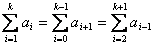Name of index and range is not always from 1 or from 0, it can start from any number. Index is dummy name. The name is not important; you can put any name as long as they are consistent.

## Example

If we have a sequence of numbers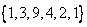then we can put index on the sequence such that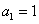,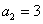,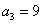,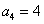,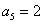,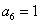and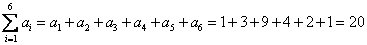But you can also start the index from zero if you like such that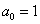,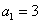,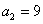,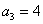,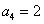,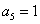and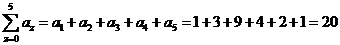Of course, the results are the same because actually they sum over the same sequence of numbers.

< Previous | Next | Contents >